Also called Gauss-Laguerre Quadrature or Laguerre Quadrature. A Gaussian Quadrature over the interval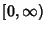with Weighting Function. The Abscissas for quadrature orderare given by the Roots of the Laguerre Polynomials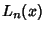. The weights are(1)

whereis the Coefficient ofin. For Laguerre Polynomials,(2)

whereis a Factorial, so(3)(4)

so(5)

(Note that the normalization used here is different than that in Hildebrand 1956.) Using the recurrence relation(6)

which implies(7)

gives(8)

The error term is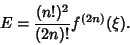(9)

Beyer (1987) gives a table of Abscissas and weights up to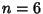.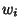2 0.585786 0.853553 3.41421 0.146447 3 0.415775 0.711093 2.29428 0.278518 6.28995 0.0103893 4 0.322548 0.603154 1.74576 0.357419 4.53662 0.0388879 9.39507 0.000539295 5 0.26356 0.521756 1.4134 0.398667 3.59643 0.0759424 7.08581 0.00361176 12.6408 0.00002337

The Abscissas and weights can be computed analytically for small.2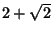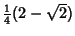For the associated Laguerre polynomial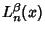with Weighting Function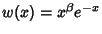,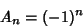(10)

and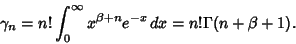(11)

The weights are(12)

whereis the Gamma Function, and the error term is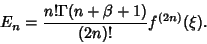(13)

References

Beyer, W. H. CRC Standard Mathematical Tables, 28th ed. Boca Raton, FL: CRC Press, p. 463, 1987.

Chandrasekhar, S. Radiative Transfer. New York: Dover, pp. 64-65, 1960.

Hildebrand, F. B. Introduction to Numerical Analysis. New York: McGraw-Hill, pp. 325-327, 1956.